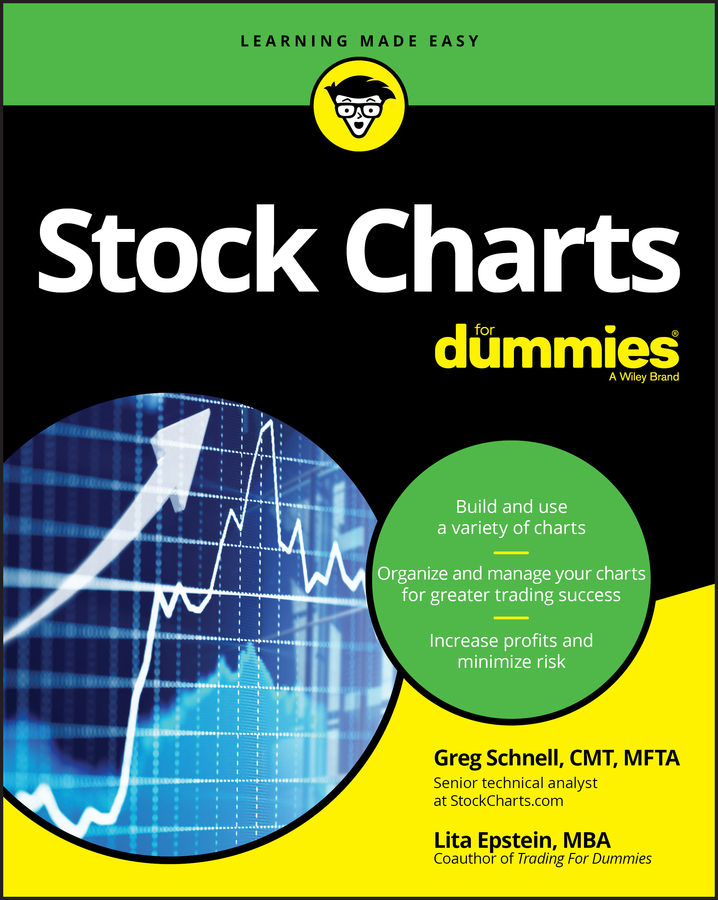##### Stock Charts For DummiesIn terms of stock price data, a favorite tool of the technical analyst is the moving average. A moving average is the average price of a stock over a set period of time (which can range from 5 days to six months — or sometimes longer). It’s considered a lagging indicator.

Frequently a chart shows price movements as too jumpy and haphazard, so the moving average smoothes out the price movements to show a clearer path. This technique helps to decipher the stock’s trend and plot out the support and resistance levels.

Moving averages are also very helpful in identifying all the various peaks and troughs necessary to analyze the trend’s direction. There are three types of moving averages: simple, linear, and exponential.

## Simple moving averages (SMA)

The first (and most common) type of average is referred to as a simple moving average (SMA). You calculate it by simply taking the sum of all the past closing prices over the chosen time period and dividing the result by the number of prices used in the calculation. For example, in a ten-day simple moving average, the last ten closing prices are added together and then divided by ten.

Say that the prices for the last ten trading days (in order) are \$20, \$21, \$22, \$20, \$21, \$23, \$24, \$22, \$22, and \$24. It’s hard to derive a trend from that data, but a moving average can help. First you add up all the prices; in this case the total is \$219.

Then you take the total of \$219 and divide it by ten (the total number of trading days). You get an average price of \$21.90. As you do this with more and more price data (in ten-day chronological sets), you can see a trend unfolding.

Say that on the 11th day, the closing price is \$26. At this point, the next ten-day trading period starts with \$21 (the closing price from the second day in the example from the preceding paragraph) and ends with a new closing price for the 10th day, \$26.

Now when you add up this new ten-day range, you get a total of \$225. When you divide that number by ten, you get the average of \$22.50 (\$225 total divided by 10 days). In this brief and simple example, the 10-day moving average tells you that the price trend is up (from \$21.90 to \$22.50).

Of course, you need to see a much longer string of ten-day sets to ascertain a useful ten-day moving average, but you get the point. These averages can also be plotted on a graph to depict the trend and help render a trading decision. The more time periods you graph, the easier it is to see how strong (or weak) the trend is.

Technical analysts most frequently use 10-day, 20-day, and 50-day averages for short-term trading. To confirm longer-term trends, they also watch the 100-day and 200-day moving averages. Of course, other time frames are used as well, but these are the most common.

The longer-period moving averages help to put the short terms in perspective so that the trader can still view the big picture. In other words, you may have a stock “correct” or pull back and see its price fall significantly, but does it mean that a trend has reversed?

If the stock is in a long-term bull market, it’s common for it to violate (or go below) its short-term (such as 10-, 20- or 50-day) moving averages temporarily. The more serious red flags start to appear when it violates the longer-term averages, such as the 200-day moving average.

## Linear and exponential averages

Some critics believe that the SMA is too limited in its scope and therefore not as useful as it should be. Therefore, they use more involved variants of the SMA such as the linear weighted average (LWA) and the exponential moving average (EMA). These averages are too involved to adequately cover here. For beginners, though, the SMA is sufficient.